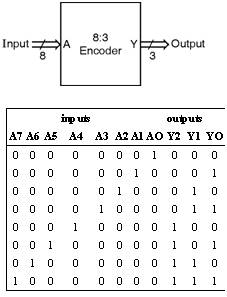Home > VHDL > Logic Circuits > 8 3 Binary Encoder

8:3 Binary Encoder :

An 8:3 encoder truth table and figure is shown below.Using if statement :

library IEEE;

use IEEE.STD_LOGIC_1164.all, IEEE.NUMERIC_STD. all;

entity ENCODER8 is

port (A: in std_logic_vector (7 downto 0);

Y: out std_logic_vector (2 downto 0));

end ENCODER8;

architecture ARCH of ENCODER8 is

begin

process (A)

begin

If (A = "00000001") then Y <= "000";

elsif (A = "00000010") then Y <= "001";

elsif (A = "00000100") then Y <= "010";

elsif (A = "00001000") then Y <= "011";

elsif (A = "00010000") then Y <= "100";

elsif (A = "00100000") then Y <= "101";

elsif (A = "01000000") then Y <= "110";

elsif (A = "10000000") then Y <= "111";

else Y <= "XXX";

end if ;

end process ;

end ARCH;

2) Using case statement :

library IEEE;

use IEEE.STD_LOGIC_1164.all, IEEE.NUMERIC_STD. all;

entity ENCODER8 is

port (A: in std_logic_vector (7 downto 0);

Y: out std_logic_vector (2 downto 0));

end ENCODER8;

architecture ARCH of ENCODER8 is

begin

process (A)

begin

case A is

when "00000001" => Y <= "000";

when "00000010" => Y <= "001";

when "00000100" => Y <= "010";

when "00001000" => Y <= "011";

when "00010000" => Y <= "100";

when "00100000" => Y <= "101";

when "01000000" => Y <= "110";

when "10000000" => Y <= "111";

when others => Y <= "XXX";

end case ;

end process ;

end ARCH;

3) Using select signal assignment :

library IEEE;

use IEEE.STD_LOGIC_1164.all, IEEE.NUMERIC_STD. all;

entity ENCODER8 is

port (A: in std_logic_vector (7 downto 0);

Y: out std_logic_vector (2 downto 0));

end ENCODER8;

architecture ARCH of ENCODER8 is

begin

with A select

Y <= "000" when "00000001",

"001" when "00000010",

"010" when "00000100",

"011" when "00001000",

"100" when "00010000",

"101" when "00100000",

"110" when "01000000",

"111" when "10000000",

"XXX" when others;

end ARCH;

4) Using conditional signal assignment :

library IEEE;

use IEEE.STD_LOGIC_1164.all, IEEE.NUMERIC_STD. all;

entity ENCODER8 is

port (A: in std_logic_vector (7 downto 0);

Y: out std_logic_vector (2 downto 0));

end ENCODER8;

architecture ARCH of ENCODER8 is

-- Conditional signal assignment equivalent to if but is concurrent so must be outside a process

begin

Y<= "000" when A = "00000001" else

"001" when A = "00000010" else

"010" when A = "00000100" else

"011" when A = "00001000" else

"100" when A = "00010000" else

"101" when A = "00100000" else

"110" when A = "01000000" else

"111" when A = "10000000" else

"XXX";

end ARCH;

5) Using for loop statement :

library IEEE;

use IEEE.STD_LOGIC_1164.all, IEEE.NUMERIC_STD. all;

entity ENCODER8 is

port (A: in unsigned (7 downto 0);

Y: out unsigned (2 downto 0));

end ENCODER8;

architecture ARCH of ENCODER8 is

begin

process (A)

variable N: integer range 0 to 7;

variable Test: unsigned(7 downto 0);

begin

Test := "00000001";

Y <= "XXX";

for N in 0 to 7 loop

if (A = Test) then

Y <= To_unsigned(N, 3); -- Loop integer converted to type unsigned for output

exit ;

end if ;

Test := shift_left(Test,1);

end loop ;

end process ;

end ARCH;